UPSC  >  Circle, Ellipse, Parabola & Hyperbola: Solved Examples

# Circle, Ellipse, Parabola & Hyperbola: Solved Examples - CSAT Preparation - UPSC

 Table of contentsThe Standard Form of CircleThe EllipseThe ParabolaThe Hyperbola## The Standard Form of Circle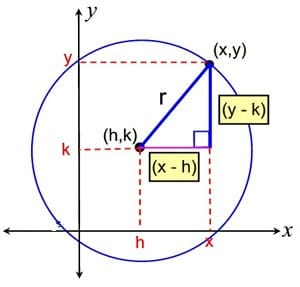• (x - h)2 + (y - k)2 = r2
Where the center is (h, k) and radius is ‘r’.
• If center of the circle is at the origin and the radius is 'r', then the equation of the circle is:
x2 + y2 = r2
• This is also known as the simplest form.

Example 1: If the area of the circle shown below is kπ, what is the value of k?
(a) 4
(b) 16
(c) 32
(d) 20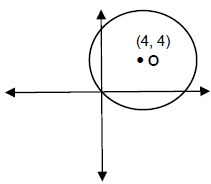Since the line segment joining (4, 4) and (0, 0) is a radius of the circle:
r2 = 42 + 42 = 32
Therefore, area = πr2 = 32π ⇒ k = 32

Question for Circle, Ellipse, Parabola & Hyperbola: Solved Examples
Try yourself:What is the coordinates of the centre and the radius of the circle with equation:
(x - 4)﻿2﻿ + (y - 3)﻿2﻿ = 25﻿﻿﻿

## The Ellipse

• Though not so simple as the circle, the ellipse is nevertheless the curve most often "seen" in everyday life. The reason is that every circle, viewed obliquely, appears elliptical.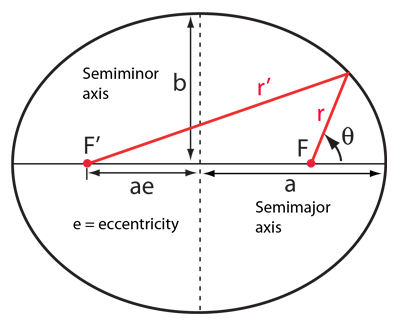Ellipse
• The equation of an ellipse centered at the origin and with an axial intersection at (±, a, 0) and (± b, 0) is: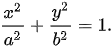## The Parabola

• When a baseball is hit into the air, it follows a parabolic path. There are all kinds of parabolas, and there’s no simple, general parabola formula for you to memorize.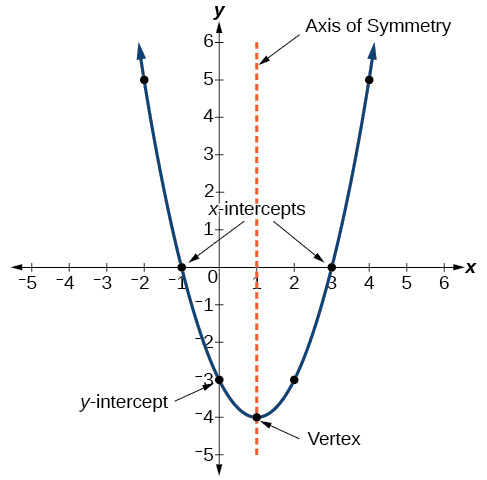Parabola
• You should know, however, that the graph of the general quadratic equation:
y = ax2 + bx + c is a parabola.
• It’s one that opens up either on top or on the bottom, with an axis of symmetry parallel to the y-axis. The graph of the general quadratic equation y = ax2 + bx + c is a parabola.
Examples: y = x2 - 2x + 1 and y = - x2 - 4 are examples of some parabolic equations.

## The Hyperbola

• If a right circular cone is intersected by a plane parallel to its axis, part of a hyperbola is formed.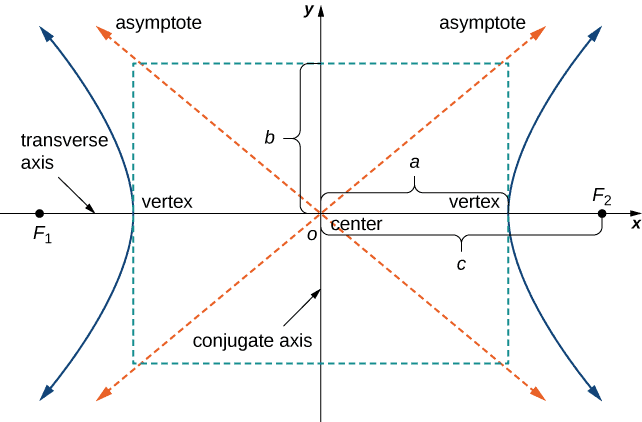Hyperbola
• The equation of a hyperbola at the origin and with foci on the x-axis is: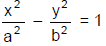Example 2: Find the area enclosed by the figure | x | + | y | = 4.

The four possible lines are:
x + y = 4; x - y = 4; - x - y = 4 and -x + y = 4
The four lines can be represented on the coordinates axes as shown in the figure. Thus a square is formed with the vertices as shown. The side of the square is: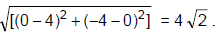The area of the square is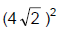= 32 sq. units.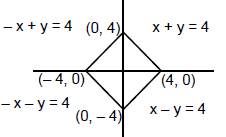Example 3: If point (t, 1) lies inside circle x2 + y2  = 10, then t must lie between:

As (t, 1) lies inside the circle, so its distance from centre i.e. origin should be less than radius i.e.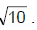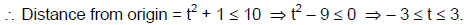Example.4 Find the equation of line passing through (2, 4) and through the intersection of line 4x - 3y - 21 = 0 and 3x - y - 12 = 0?

4x - 3y - 21 = 0 …..(1)
3x - y - 12 = 0 ….(2)
Solving (1) and (2), we get point of intersection as x = 3 & y = - 3.
Now we have two points (3, -3) & (2, 4)
⇒ Slope of line m =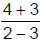= - 7
So, the equation of line is:
⇒ y + 3 = - 7 (x - 3)
⇒ 7x + y - 18 = 0
Alternate Method:
Equation of line through intersection of 4x - 3y - 21 = 0 and 3x - y - 12 = 0 is:
(4x - 3y - 21) + k(3x - y - 12) = 0.
As this line passes through (2, 4):
⇒ (4 × 2 - 3 × 4 - 21) + k(3 × 2 - 4 - 12) = 0
⇒ k =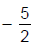So, the equation of line is: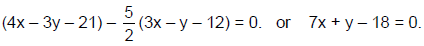The document Circle, Ellipse, Parabola & Hyperbola: Solved Examples | CSAT Preparation - UPSC is a part of the UPSC Course CSAT Preparation.
All you need of UPSC at this link: UPSC

## CSAT Preparation

197 videos|151 docs|200 tests

## FAQs on Circle, Ellipse, Parabola & Hyperbola: Solved Examples - CSAT Preparation - UPSC

 1. What is the standard form of a circle?Ans. The standard form of a circle is (x - h)^2 + (y - k)^2 = r^2, where (h, k) represents the center of the circle and r represents the radius.
 2. How is an ellipse different from a circle?Ans. An ellipse is different from a circle in terms of its shape. While a circle has all points equidistant from its center, an ellipse has two different radii, known as major and minor axes, resulting in a stretched or compressed circular shape.
 3. What is the equation of a parabola?Ans. The equation of a parabola in its standard form is y = ax^2 + bx + c, where a, b, and c are constants. The parabola can open upwards or downwards depending on the value of "a".
 4. How can you distinguish between a hyperbola and an ellipse?Ans. A hyperbola and an ellipse can be distinguished by the sum of the distances from any point on the curve to two fixed points called foci. In an ellipse, the sum of distances is constant, while in a hyperbola, the difference of distances is constant.
 5. How can the standard form of each conic section be used in real-life applications?Ans. The standard form of each conic section (circle, ellipse, parabola, hyperbola) is used in various real-life applications such as satellite orbits (ellipse), telescope mirrors (parabola), architectural designs (circle), and radio wave antennas (hyperbola). These forms provide precise mathematical representations for these shapes, allowing engineers and designers to optimize their designs.

## CSAT Preparation

197 videos|151 docs|200 testsExplore Courses for UPSC exam### How to Prepare for UPSC

Read our guide to prepare for UPSC which is created by Toppers & the best Teachers
Signup to see your scores go up within 7 days! Learn & Practice with 1000+ FREE Notes, Videos & Tests.
10M+ students study on EduRev
Track your progress, build streaks, highlight & save important lessons and more!
Related Searches

,

,

,

,

,

,

,

,

,

,

,

,

,

,

,

,

,

,

,

,

,

,

,

,

,

,

,

;